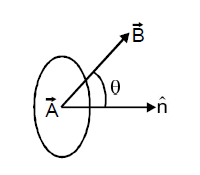# Magnetic Flux

Consider a closed curve enclosing an area A (as shown in the figure). Let there be a uniform magnetic field B in that region. The magnetic flux through the area A is given byΦ = B.A = B A CosΘ

where Θ is the angle which the vector B makes with the normal to the surface. If B is perpendicular to A, then the flux through the closed area A is zero.

SI unit of magnetic flux is weber (Wb).

## Notes :

• Area vector is ⊥ to the surface.
• For open surface choose one direction as the area vector direction and stick to it for the whole problem.
• For closed surfaces outward normal is taken as area vector direction
• Flux is basically count of number of lines crossing a surface
•[because magnetic field lines exists in closed loop.]

Announcements

Join our Online JEE Test Series for 499/- Only (Web + App) for 1 Year

Join our Online NEET Test Series for 499/- Only for 1 Year